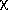D.9 Conversion of Position Uncertainties to Gaussian Approximation

# IRAS Explanatory Supplement V. Data Reduction D. Point Source Confirmation D.9 Conversion of Position Uncertainties to Gaussian Approximation

Chapter Contents | Introduction | Authors | References
The error bars were obtained as follows. For Gaussian errors, the two-dimensional 95% confidence would correspond to a2 probability of 0.95 with two degrees of freedom. This implies a value of 5.99 for the2 random variable, so that the eclipse crosses each axis at 2.45-, corresponding to a confidence of 98.6%. This was used for the confidence level for both axes. On the Gaussian axis of the error model, the interval used was 2.45-y. On the non-Gaussian axis, a lookup table was used to obtain the 98.6% confidence deviation as a function of the ratio of the uniform half-width, Lz, to the Gaussianz. This table was computed by numerical quadrature for a grid of these ratios ranging from 0.1 to 30. Below 0.1, the probability density was considered Gaussian, and above 30 it was taken as purely uniform.